Case Based Questions (MCQ)

Chapter 4 Class 10 Quadratic Equations
Serial order wise

## Raj and Ajay are very close friends. Both the families decide to go to Ranikhet by their own cars. Raj’s car travels at a speed of x km/h while Ajay’s car travels 5 km/h faster than Raj’s car. Raj took 4 hours more than Ajay to complete the journey of 400 km.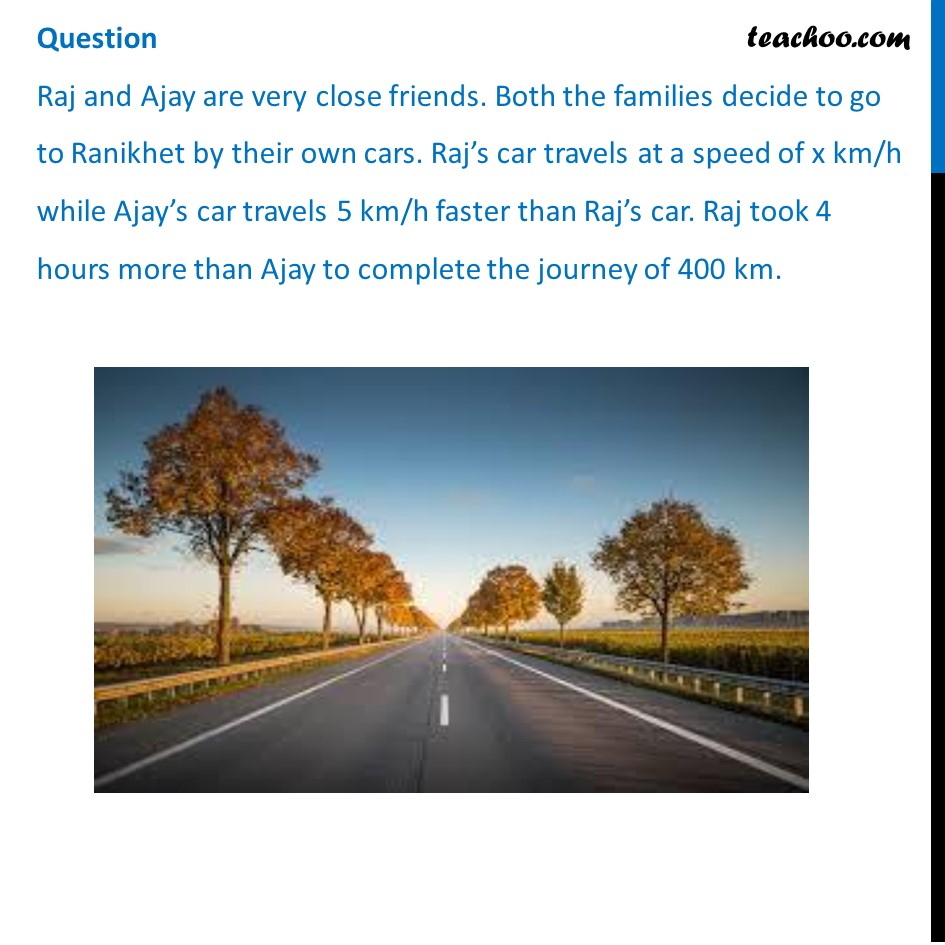## What will be the distance covered by Ajay’s car in two hours? (a) 2(x + 5) km (b) (x - 5) km (c) 2(x + 10) km (d) 2(x + 5) km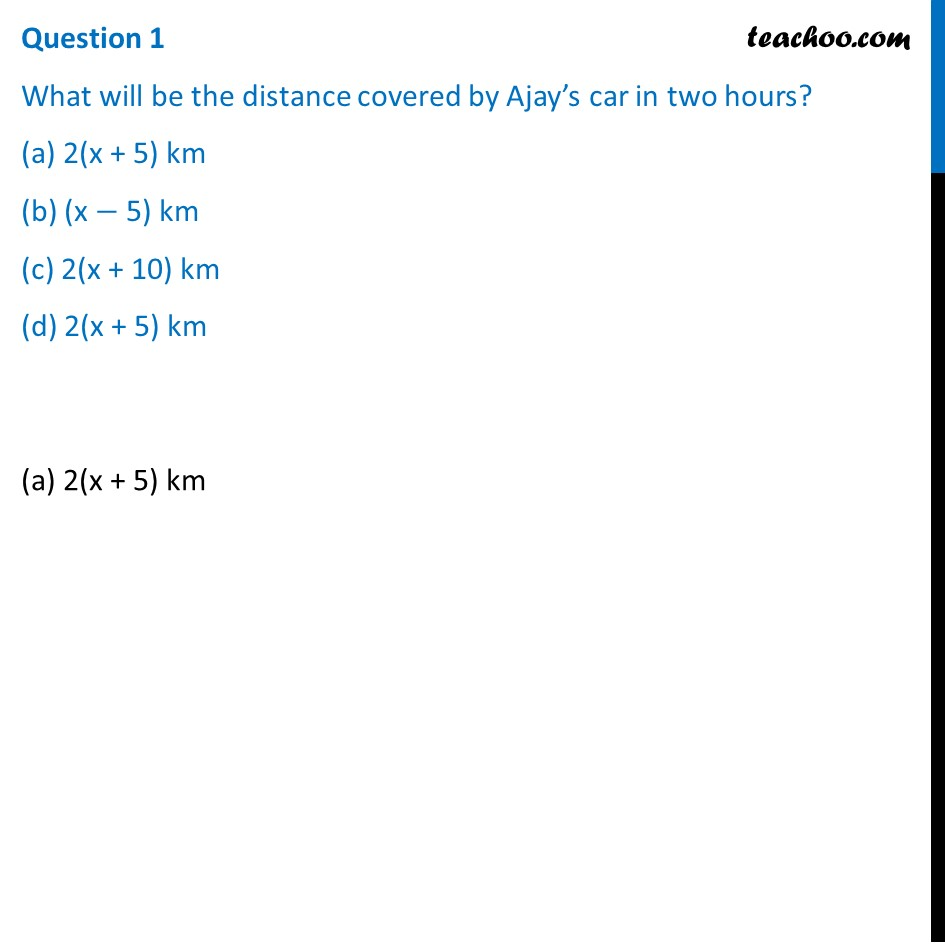## Which of the following quadratic equation describe the speed of Raj’s car? (a) x 2 -5x-500=0  (b) x 2 - 4x-400=0   (c) x 2 +5x-500=0   (d) x 2 -4x+400=0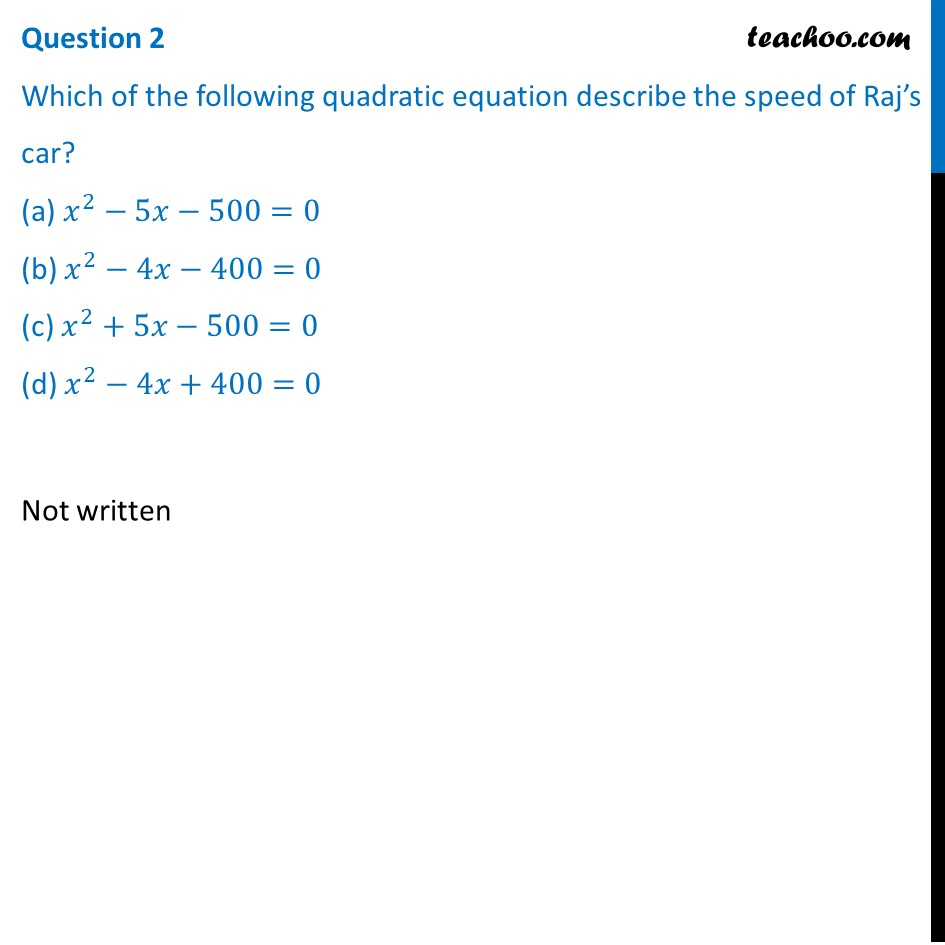## What is the speed of Raj’s car? (a) 20 km/hour (b) 15 km/hour (c) 25 km/hour (d) 10 km/hour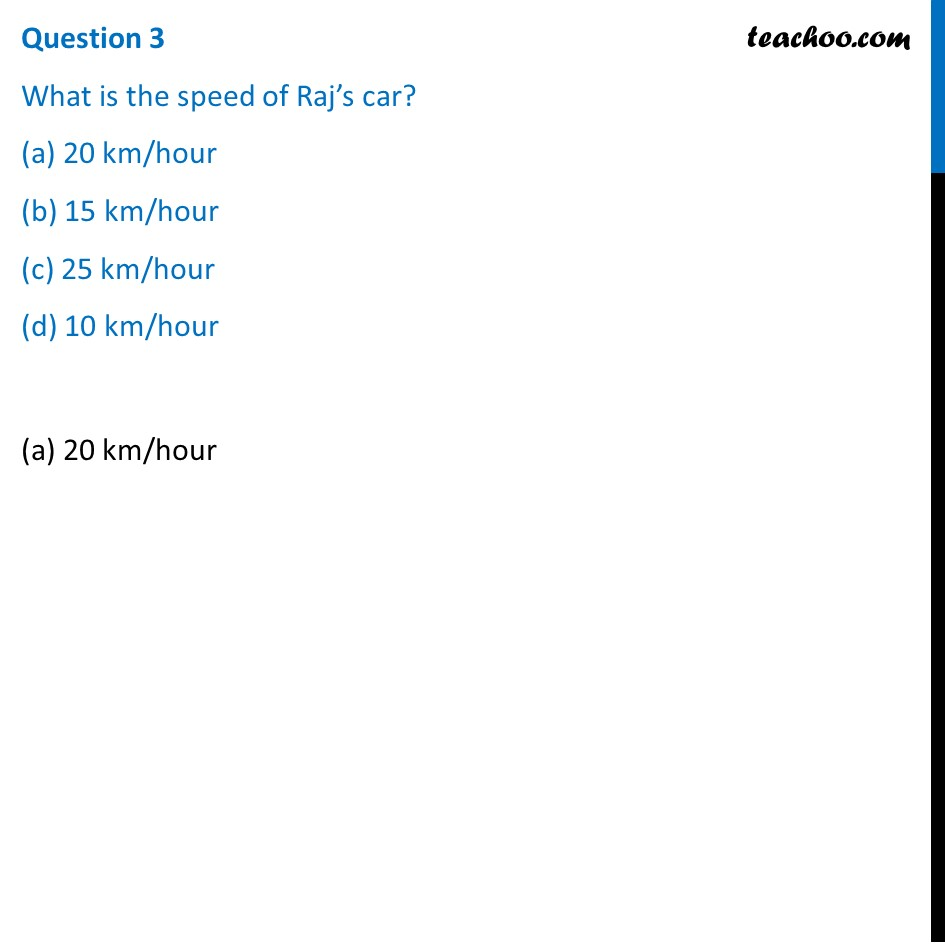## How much time took Ajay to travel 400 km? (a) 20 hour (b) 40 hour (c) 25 hour (d) 16 hour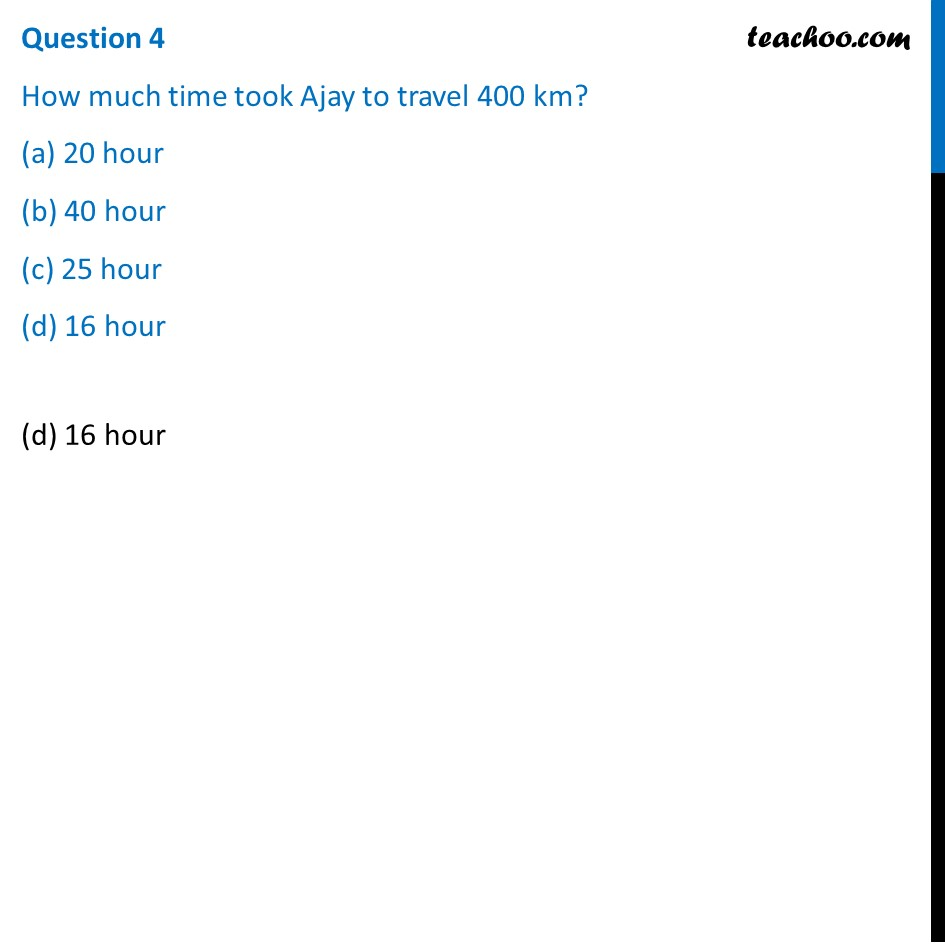Learn in your speed, with individual attention - Teachoo Maths 1-on-1 Class

### Transcript

Question Raj and Ajay are very close friends. Both the families decide to go to Ranikhet by their own cars. Raj’s car travels at a speed of x km/h while Ajay’s car travels 5 km/h faster than Raj’s car. Raj took 4 hours more than Ajay to complete the journey of 400 km.Question 1 What will be the distance covered by Ajay’s car in two hours? (a) 2(x + 5) km (b) (x − 5) km (c) 2(x + 10) km (d) 2(x + 5) km(a) 2(x + 5) km Question 2 Which of the following quadratic equation describe the speed of Raj’s car? (a) 𝑥^2−5𝑥−500=0 (b) 𝑥^2−4𝑥−400=0 (c) 𝑥^2+5𝑥−500=0 (d) 𝑥^2−4𝑥+400=0 Not written Question 3 What is the speed of Raj’s car? (a) 20 km/hour (b) 15 km/hour (c) 25 km/hour (d) 10 km/hour (a) 20 km/hour Question 4 How much time took Ajay to travel 400 km? (a) 20 hour (b) 40 hour (c) 25 hour (d) 16 hour(d) 16 hour